# Letters as Numbers

We use letters in math to represent numbers. We do this mostly for two reasons:

We know the meaning of the number, but we don’t know the value (unknown).

Eg, the number of cards I will sell at the craft fair tomorrow. Suppose the cards cost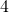dollars each, I can calculate the amount of money I will make by letting the number of cards I sell be represented by a letter, say ‘n’. I will makedollars.

We know the meaning of the number and the number changes (variable).

Eg, My heartrate during a one hour workout at the gym. I know that my heartrate will be between 50 beats per minute at 180 beats per minute. My heartratewill vary between 50 and 180 during the workout. At any moment during the workout, I can check my heart rate monitor to see what my heartrate is exactly.

# Definitions

A term is a single number or unknown (a number represented by a letter), or the product (multiplication) of a number with unknowns. If the unknowns become known, the value of the term can be calculated. Terms can be positive or negative.

Here are four terms: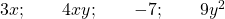Suppose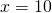. Then the value of the termis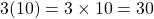.

An expression is the addition of one or more terms. If the unknowns become known the value of the expression can be calculated.

Here is an expression made by adding four terms together (note that adding a negative is the same as subtracting):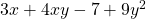This expression has two unknowns, represented byand.

Writing two unknowns together means to multiply them, egmeans.

If it is given thatand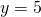, the value of this expression is: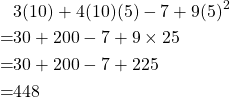Note that this expression can be written in any order because addition is commutative:A linear expression is an expression that includes a constant or an unknown or both. The unknown has power one. When we draw a graph of a linear expression (linear relation), the graph is a straight line. This is why it is called ‘linear’.

Here are four linear expressions:.

# Simplify An Expression

We simplify an expression when we collect like terms together. This is kind of like organising the cutlery drawer, or counting a jar full of coins.https://farm2.static.flickr.com/1431/566441814_6004fe8712_b.jpg

When sorting the coins, we generally sort by the type of coin. E.g. put all the loonies together, the toonies together etc to simplify the final calculation.

What counts as ‘simple’ can be debated. It really depends on the context. The skills to have to hand are the following:

• gather like terms;
• expand brackets;
• factor a common factor.

# Gather like terms

We gather like terms to reduce the number of terms an expression has. This is just like combining all the forks together and all the knives together, except that we are gathering like numbers. Remember that a letter is there to represent a number that we might know the meaning of but not the value of.

Example 1:.

Note that when a letter appears without a number (coefficient), the number is 1.

Example 2:.

When we have two or more different letters or different combinations of letters, we remember that different letters stand for different numbers. We can’t gather different letters together.

Example 3:.

Constants state their own value. We can gather constants together.

Example 4:.

Number combinations need to stick together:

Example 5: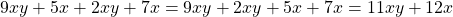Try it out here:

applet

# Expanding brackets

We use brackets (parenthesis) when we need to multiply a whole expression by a number or by another expression.

Let’s take the linear expression, and multiply the whole thing by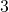.

If we simply sayit looks like we are multiplyingby 3 and poor 4 is left behind. That’s why we need brackets.

Remember that multiplication is shorthand for repeated addition so let’s decode:.

The distributive property lets us do this more efficiently:

The distributive property states that.

In other words,Try it out here:

applet

# Factor

To factor a linear expression is to reverse the ‘expand the brackets’ process. To do it we look for a common factor in each term.

We say ‘factor fully’ to make sure we take the greatest common factor from each terms.Try it out here:

applet

We do a lot more factoring in Unit 3.  We factor numbers using prime numbers. We then factor expressions with unknowns that use exponents. We also factor the product (multiple) of two linear expressions – this is called a quadratic expression or a trinomial.

# Keep the Value

The value of the expression before any process must be the same as the value after the process. We can test this by using any number for the unknown.

Consider the expression.

Suppose,.

Now, lets factor the expression.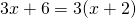Now, leton the new expression,.  We have,as before.

Therefore, the value of the expression has not been altered during this process.

# Expand, Simplify, Factor

Simplifying is an important part of handling algebra. To simplify an expression makes our work as we go makes our math more efficient and reduces the errors we might make.

Check out the old equations on this page. Solving equations becomes more straightforward when we simplify sides before beginning the solving process. Remember that simplifying changes how an expression appears (looks) but not its value.